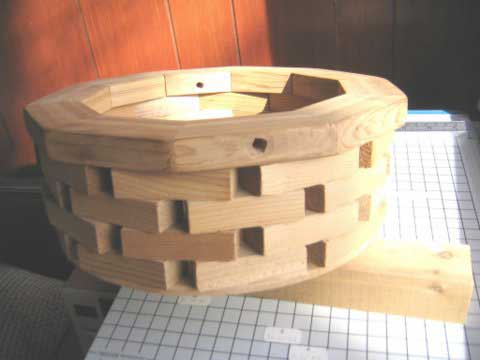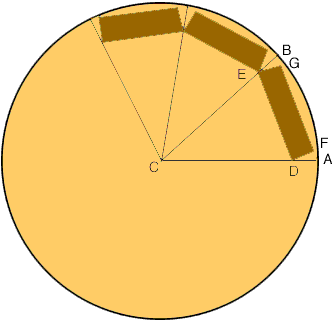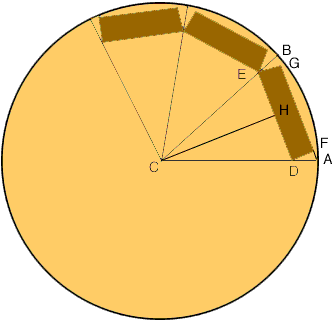Quandaries and Queries I am building a wishing-well out of pieces of 2-by-4. I have included a picture of a miniature version of what I want. There are to be ten 2-by-4 pieces around the well and I want the circle around the outside of the structure to have a diameter of approximately 3 feet. How long to I cut the 2-by-4's to build the wishing-well. Thanks, Don Hi Don, Here is the photo you included.I sketched a top-down view of the first row of 2-by-4's on a circular base of diameter 3 feet and labeled some points. (The diagram is not to scale.)Since there are 10 pieces of 2-by-4 and the angle at the center of a circle is 360o, the angle BCA is 36o. Now bisect the angle BCA and extend the side GF of the 2-by-4 to meet the outside circle at A.The line segments CH and DF are parallel and the angle HCD is 18o, hence the angle FDA is 18o. Angle DFA is a right angle and hence cos( 18o) = |FD|/|DA| or |DA| = |FD|/cos(18o) The width of a 2-by-4 is 3.5 inches so |DA| = 3.5/0.9511 = 3.68 inches. Thus |CD| = 18 - 3.68 = 14.32 inches. Now look at triangle HCD. Angle HCD is 18o, and sin( 18o) = |HD|/|CD| so |HD| = |CD|sin(18o) = 14.323.309 = 4.43 inches. Thus the length of the 2-by-4 is 24.43 = 8.83 inches. This is approximately 8 and 7/8 inches. Harley Go to Math Central